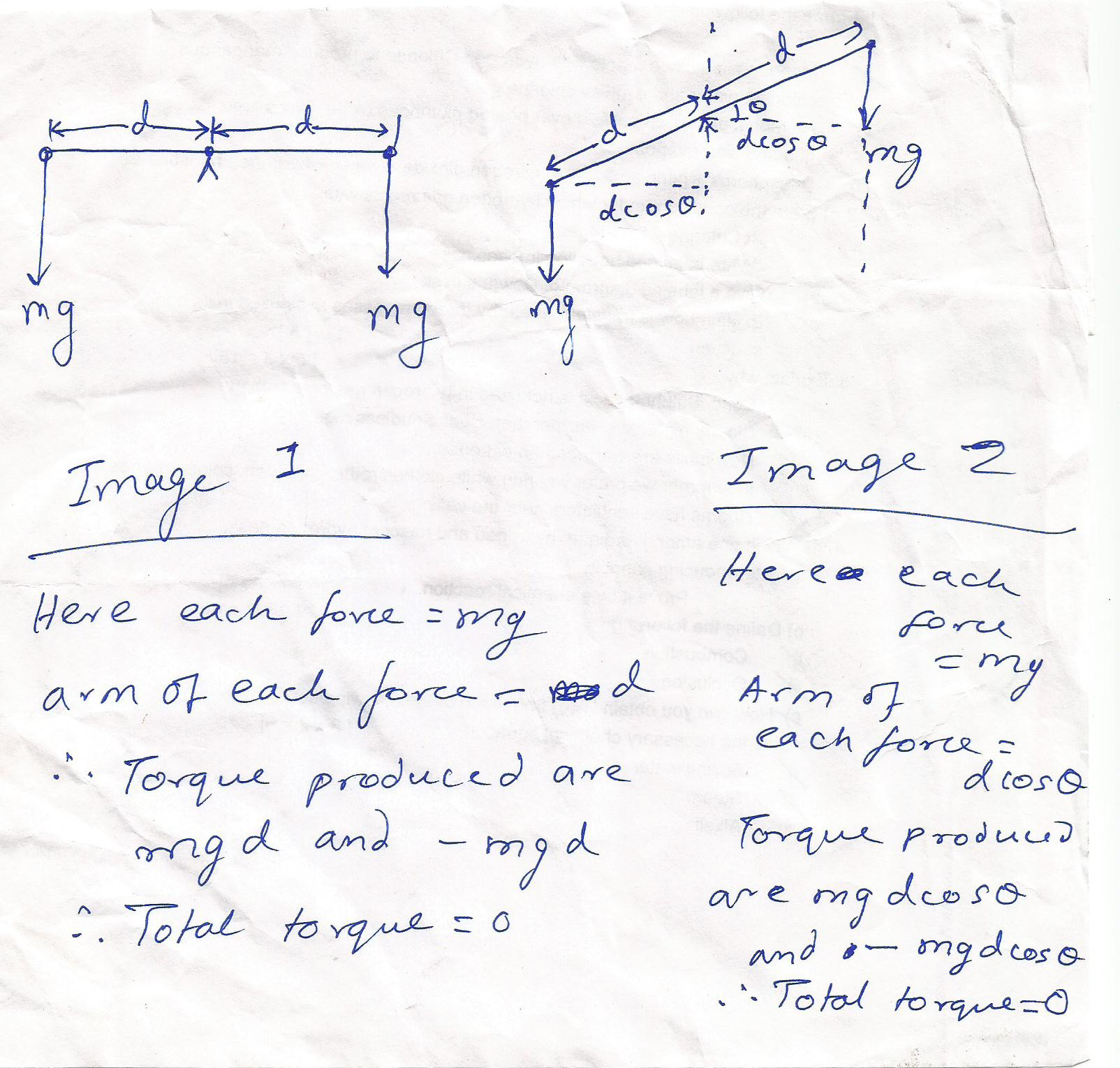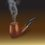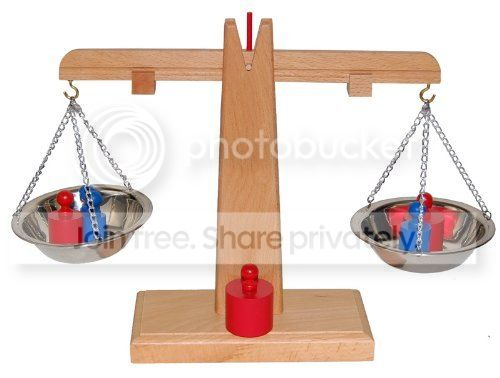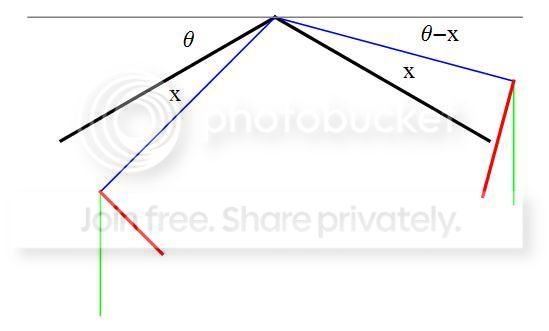# The Physical Balance ProblemWe all know the mechanism of physical balance. The balance is supported at the center and two masses are hung from the two ends. The masses being in the earth's gravitational field (which can be justifiably considered to be an uniform field acting vertically downwards at such a small scale), produce torques which are opposite to each other in direction. If the torques are equal in magnitude then the mass dipole (we can consider the balance beam simply a mass dipole) is at the equilibrium condition, i.e., perpendicular to the earth's gravitational field. If we additionally assume that the arm-lengths of the balance are equal then the above situation assures us that the counterpoised weights and hence masses are equal. Image 1 illustrates this situation. However the real problem is yet to come. Suppose a mischievous experimenter slightly displaces the balance beam from its equilibrium position making it no longer perpendicular to the field. Now as shown in Image 2, the downward acting forces are still equal(both are still mg, as the figure shows). And so are the arms of the forces about the axis of rotation(they no longer have their previous value though, but still, they are equal). That means the torques produced are still equal and opposite. So, my question is, why does the balance come down to its original equilibrium position as soon as it is released? From where does it get this torque to rotate back to its original configuration? Think and answer..;-)Note by Kuldeep Guha Mazumder
6 years ago

This discussion board is a place to discuss our Daily Challenges and the math and science related to those challenges. Explanations are more than just a solution — they should explain the steps and thinking strategies that you used to obtain the solution. Comments should further the discussion of math and science.

When posting on Brilliant:

• Use the emojis to react to an explanation, whether you're congratulating a job well done , or just really confused .
• Ask specific questions about the challenge or the steps in somebody's explanation. Well-posed questions can add a lot to the discussion, but posting "I don't understand!" doesn't help anyone.
• Try to contribute something new to the discussion, whether it is an extension, generalization or other idea related to the challenge.

MarkdownAppears as
*italics* or _italics_ italics
**bold** or __bold__ bold
- bulleted- list
• bulleted
• list
1. numbered2. list
1. numbered
2. list
Note: you must add a full line of space before and after lists for them to show up correctly
paragraph 1paragraph 2

paragraph 1

paragraph 2

[example link](https://brilliant.org)example link
> This is a quote
This is a quote
    # I indented these lines
# 4 spaces, and now they show
# up as a code block.

print "hello world"
# I indented these lines
# 4 spaces, and now they show
# up as a code block.

print "hello world"
MathAppears as
Remember to wrap math in $$ ... $$ or $ ... $ to ensure proper formatting.
2 \times 3 $2 \times 3$
2^{34} $2^{34}$
a_{i-1} $a_{i-1}$
\frac{2}{3} $\frac{2}{3}$
\sqrt{2} $\sqrt{2}$
\sum_{i=1}^3 $\sum_{i=1}^3$
\sin \theta $\sin \theta$
\boxed{123} $\boxed{123}$

Sort by:

I've got it! General Relativity! Gravity and warped spacetime!Pix of cheap balance

- 6 years ago

What, relativity?

- 5 years, 7 months ago

GENERAL Relativity

- 5 years, 7 months ago

No no..no question of gravity and warped space-time..it is preposterous even to imagine the effects of space-time warping in a daily-needs store (well, most of the local shops here in Kolkata still use physical balances).. Think simpler..

The answer to this problem lies at the center of the balance. Look that a free mass dipole never rotates in an uniform gravitational field (unlike electric or magnetic dipoles). This is simply because unlike electric charge (or magnetic poles), mass is not of dual nature. Mass is simply mass, there is nothing seen as positive mass or negative mass (at least within the purview of classical mechanics). Why do electric dipoles rotate in uniform electrostatic fields? Simply because the electrostatic forces on the two end charges of the dipole (which are of opposite nature) are anti-parallel, resulting in a couple which rotates the dipole. But this is not the case for a mass dipole. A free mass dipole always translates in an uniform gravitational field. Then why does our physical balance rotate? It is, after all a mass dipole. This is simply because it is not free, it is clamped at the center. Think and figure out the rest of the problem.

Kuldeep, it took me a while to find that picture of a cheap scale. It is so cheaply made it gives away the reason why balance scales behave the way you've described. I'm waiting to see if anybody else catches on.

Edit: Here's a website that gives more precise details on how to build an equal arm balance scale (apparently accurate enough for use in amateur rocketry)

Construction of a Simple Balance Beam Scale

If one looks at the details closely enough, one should be able to see why balance beam scales behave as they do.

- 6 years ago

That is a great insight to mass dipoles and yes they should only translate unless fixed by external constraints. But the fixed point, if is at the centre of the dipole still should produce zero net torque and hence any configuration change(rotation) about this fixed point is neutral equillibrium and hence I am unable to get why the balance behaves as it does in our everyday life/

- 3 years, 7 months ago

Consider a balance beam that is “bent” (which just means that the center support and the end points of the arms are not strictly collinear). The graphic here shows a greatly exaggerated “bent” beam, the black showing the rest position and the blue showing the same but offset angularly by $x$.The downward force by equal weights on either end of the beam are shown in green. The torque force exerted on equal blue arms are shown in red. The net torque is proportional to

$Cos\left( \theta -x \right) -Cos\left( \theta +x \right)$

which simplifies to

$2Sin\left( \theta \right) Sin\left( x \right)$

which, to a good approximation, follows Hooke’s law

$F=kx$

where $k=2Sin\left( \theta \right)$

Hence, when the balance beam is offset by small angle $x$, it will oscillate as if against a spring, and friction losses will bring it to a rest where $x=0$.

- 6 years ago

Suppose instead of a balance we take a perfectly straight mass-less rod with two equal masses at two ends and hold at its center. If then we make it offset by a small angle then also it comes back to its original position. Where does it get the torque from in this case?

I would be interested to know of an instance where something free to rotate about its center of gravity will rotate (in a divergence free field of gravity) to some other position and then stay there. A lot of motorcyclists that find themselves at a bad angle mid-air during a jump would like to know that.

- 6 years ago

Gravity is a curl-free field and not a divergence-free one..it is irrotational..it has high negative divergence..with field sinks at mass distributions..that is why we speak of a scalar and not a vector gravitational potential unlike magnetic potential which is vector (magnetic field is solenoidal)..

I'm referring to a local uniform gravitational force on all the parts of the system, i.e., not only irrotational, but in same direction with uniform magnitude. The simplest possible case "as a good approximation of the real thing"

Practical equal arm balance scales don't "right" themselves on account of the fact that gravity does typically have a [incredibly tiny local] divergence. It would take extremely sensitive instruments to be able to detect the divergence.

- 6 years ago